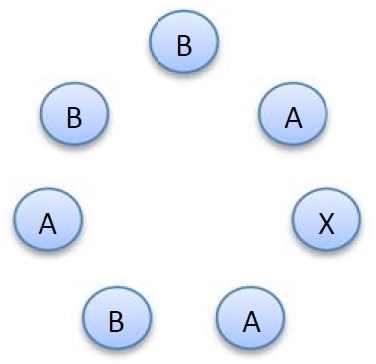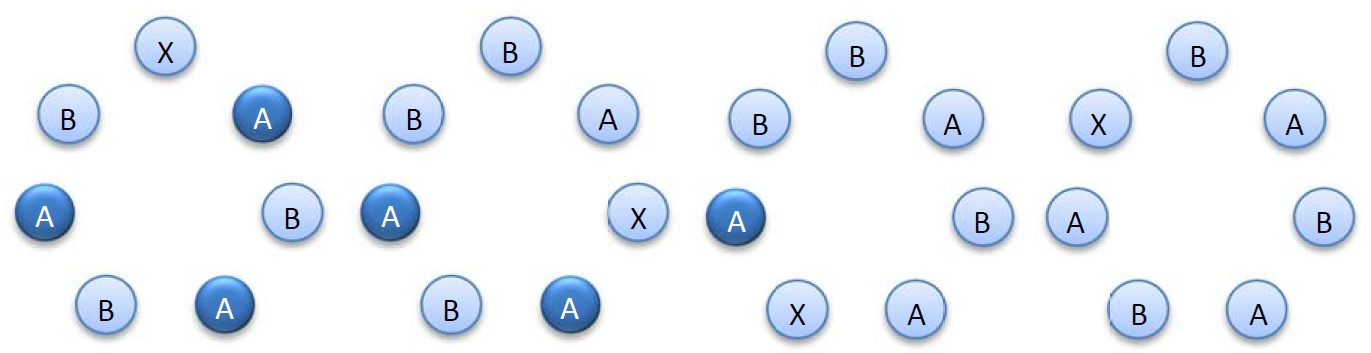# #297. 【CTSC2017】密钥1. 给定密钥中所有 A 的位置，当密钥的特征值为 $0$ 时，请问 X 在哪个位置。
2. 给定密钥中所有 A 的位置，当密钥的特征值为 $S$ 时，请问 X 在哪个位置。
3. 给定密钥中所有 B 的位置，当密钥的特征值为 $S$ 时，请问 X 在哪个位置。

### 输出格式

1. 第一个数表示当 $k$ 元集合 $P$ 代表 A 的位置且特征值为 $0$ 时 X 的位置。
2. 第二个数表示当 $k$ 元集合 $P$ 代表 A 的位置且特征值为 $S$ 时 X 的位置。
3. 第三个数表示当 $k$ 元集合 $P$ 代表 B 的位置且特征值为 $S$ 时 X 的位置。

### 样例一

#### input

5
3344
2



#### output

10
1
2



### 样例二

#### input

500000
4545
234567



#### output

999992
246922
753067



### 限制与约定

1. 如果第一问回答正确，你将获得 $3$ 分。
2. 如果第二问回答正确，你将获得 $4$ 分。
3. 如果第三问回答正确，你将获得 $3$ 分。